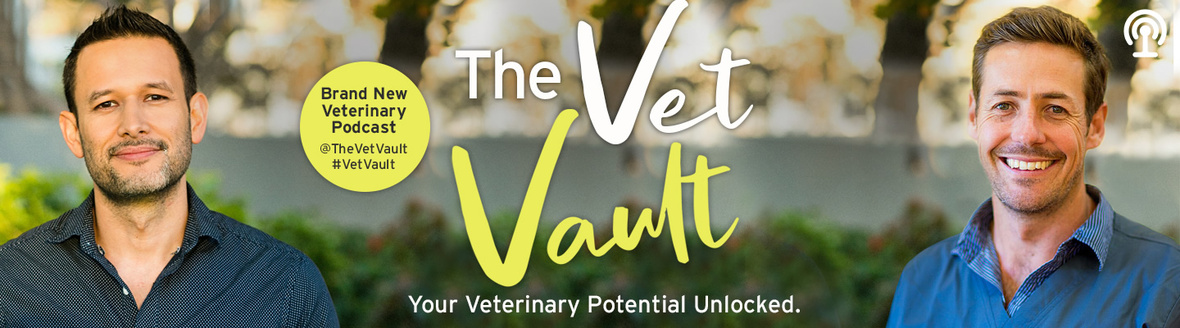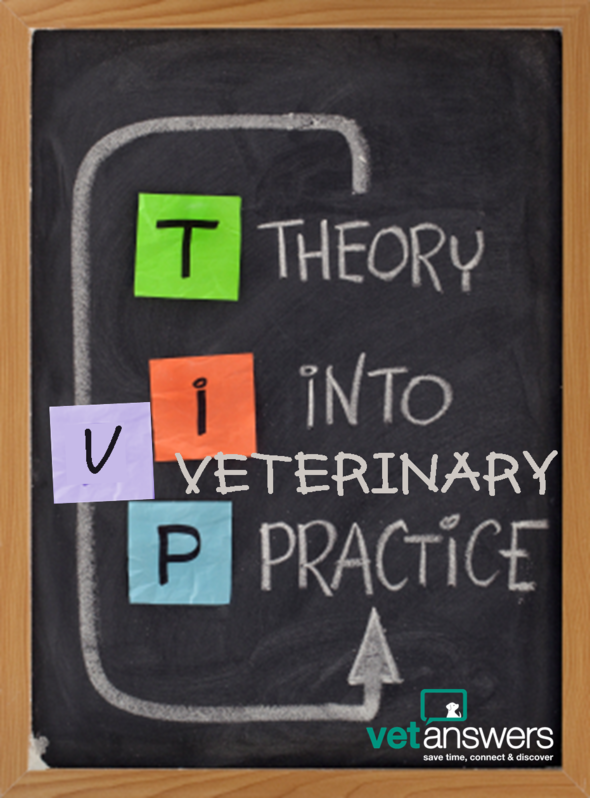Like   Tweet   Pin   in

Hi Member,

This week we welcome the next episode of our favourite Guest Blog Post series: So Many Other things: The Reality of Veterinary Practice as well as an interesting acupuncture case study - check out Kevin's Story (it really should be called: How Kevin got his groove back...with acupuncture!)

I've also recently started listening to Podcasts and have been enjoying Dr Gerardo Poli and Dr Hubert Hiemstra's The Vet Vault. They've had some great guests so far and I recommend the series to everyone - no matter what your position in the veterinary industry.

You'll also find Part 4 in a really fascinating and must-read series of posts published by ViN News 'Fixing the veterinary profession before it's too late' along with a very special offer from our friends at Vetsone.

## Save Time, Connect & Discover!table div table+table+table+table div table{width:100%;padding:0}table div table+table+table+table div table img{width:96.23%;padding:0;float:none}table div table+table+table+table div table td{width:100%;padding:0 1.88% 18px}/* styles */## So Many Other Things: The Reality of Veterinary Practice Part 11 - Rate, Risk, Care

Cosmo’s heartbreaking death continued to impact some of Brudine’s staff beyond the moments, hours and days after. Dr Charlie Webb, who has handling Cosmo’s case and who relayed the tragic events to Cosmo’s owner, was replaying the events of the day, over and over, weeks and months later....

## Acupuncture as an adjunct to treatment of lumbosacral and degenerative joint disease in a pug

Kevin is a 10-year-old male castrated pug who had always been a very agile and energetic dog. However, in August 2018 he had reached a state of not being able to run, play or go for his normal long walks anymore. Kevin was referred by his regular vet for acupuncture to see if it could relieve his symptoms and increase his quality of life.

Love Your Pet Love Your Vet aims to raise awareness of veterinarian wellbeing, reduce the stigma in seeking help and provide support to veterinary professionals. Find out more about the great work they do here...

 table div table+table+table+table+table+table+table+table+table div table{width:100%;padding:0}table div table+table+table+table+table+table+table+table+table div table img{width:96.23%;padding:0;float:none}table div table+table+table+table+table+table+table+table+table div table td{width:100%;padding:0 1.88% 18px}/* styles */# Free Listing: Clipper Blade Sharpening

Clipper Blade Sharpening professionally sharpen clipper blades and repairs clippers for the pet industry. They offer a 24hr turnaround on blades and all blades are tested & quality guaranteed.

# Free Listing: Oz Grooming World

Oz Grooming World is a specialist importer of professional animal grooming products, veterinary products, dog show equipment & K9 shutzhund gear.

 table div table+table+table+table+table+table+table+table+table+table+table+table+table div table{width:100%;padding:0}table div table+table+table+table+table+table+table+table+table+table+table+table+table div table img{width:96.23%;padding:0;float:none}table div table+table+table+table+table+table+table+table+table+table+table+table+table div table td{width:100%;padding:0 1.88% 18px}/* styles */## What's on in 2019? Veterinary CPD Calendar... Featuring:

 table div table+table+table+table+table+table+table+table+table+table+table+table+table+table+table div table td,table.module-14{width:100%;padding:0}table div table+table+table+table+table+table+table+table+table+table+table+table+table+table+table div table{width:100%;float:none;margin-left:auto;margin-right:auto;padding:0}table div table+table+table+table+table+table+table+table+table+table+table+table+table+table+table div table a{border:0 none;text-decoration:none}table div table+table+table+table+table+table+table+table+table+table+table+table+table+table+table div table img{width:100%!important;border:0 none;text-decoration:none}/* styles */
 table.module-15{width:75.28%;padding:0}table div table+table+table+table+table+table+table+table+table+table+table+table+table+table+table+table div table{width:75.28%;float:none;margin-left:auto;margin-right:auto;padding:0}table div table+table+table+table+table+table+table+table+table+table+table+table+table+table+table+table div table a{border:0 none;text-decoration:none}table div table+table+table+table+table+table+table+table+table+table+table+table+table+table+table+table div table img{width:100%!important;border:0 none;text-decoration:none}table div table+table+table+table+table+table+table+table+table+table+table+table+table+table+table+table div table td{width:100%;padding:0}/* styles */

 table div table+table+table+table+table+table+table+table+table+table+table+table+table+table+table+table+table+table div table{width:100%;padding:0}table div table+table+table+table+table+table+table+table+table+table+table+table+table+table+table+table+table+table div table img{width:96.23%;padding:0;float:none}table div table+table+table+table+table+table+table+table+table+table+table+table+table+table+table+table+table+table div table td{width:100%;padding:0 1.88% 18px}/* styles */## A great Podcast & it's not just for vets!I'm a Podcast newbie, but I think I've started off with a good one:

# The Vet Vault - Your Veterinary Potential Unlocked

Hosted By Dr Gerardo Poli and Dr Hubert Hiemstra, this Podcast series aims to deliver tips, tools and inspiration to help everyone in the veterinary profession.

The first six episodes have delivered a diverse and interesting range of guests and I'm looking forward to more.

## Guests so far include:

Dr Louisa Graham - aka louisa_the_vet (18.1k followers on Instagram)
Dr Brooke Schampers - Good attitude, great outcomes
Dr Oliver Liyou - To the brink and back: a guide to surviving and thriving in veterinary science
Dr Nadine Hamilton - Mental health masterclass
Dr Paula Parker - Simple habits, big results

Check it out by searching for The Vet Vault...table div table+table+table+table+table+table+table+table+table+table+table+table+table+table+table+table+table+table+table+table+table+table div table{width:100%;padding:0}table div table+table+table+table+table+table+table+table+table+table+table+table+table+table+table+table+table+table+table+table+table+table div table img{width:96.23%;padding:0;float:none}table div table+table+table+table+table+table+table+table+table+table+table+table+table+table+table+table+table+table+table+table+table+table div table td{width:100%;padding:0 1.88% 18px}/* styles */table div table+table+table+table+table+table+table+table+table+table+table+table+table+table+table+table+table+table+table+table+table+table+table div table td,table.module-22{width:100%;padding:0}table div table+table+table+table+table+table+table+table+table+table+table+table+table+table+table+table+table+table+table+table+table+table+table div table{width:100%;float:none;margin-left:auto;margin-right:auto;padding:0}table div table+table+table+table+table+table+table+table+table+table+table+table+table+table+table+table+table+table+table+table+table+table+table div table a{border:0 none;text-decoration:none}table div table+table+table+table+table+table+table+table+table+table+table+table+table+table+table+table+table+table+table+table+table+table+table div table img{width:100%!important;border:0 none;text-decoration:none}/* styles */
 table div table+table+table+table+table+table+table+table+table+table+table+table+table+table+table+table+table+table+table+table+table+table+table+table+table div table{width:100%;padding:0}table div table+table+table+table+table+table+table+table+table+table+table+table+table+table+table+table+table+table+table+table+table+table+table+table+table div table img{width:96.23%;padding:0;float:none}table div table+table+table+table+table+table+table+table+table+table+table+table+table+table+table+table+table+table+table+table+table+table+table+table+table div table td{width:100%;padding:0 1.88% 18px}/* styles */# Is today's approach to veterinary education outdated?

## By Michael Dicks & Melissa Maddux

This week the 4th in the series of posts by economist Michael Dicks, discussing why he believes the veterinary profession isn't performing to the expectations of those involved and suggesting strategies for improvement.

In the fourth post, Dicks discusses increasing student debt. Although based on USA student costs there are still valid questions:
Faced with declining funding, should universities increase the amount charged per student or should it accept more students to share the burden?
Neither option is appealing and coming up with better solutions needs all involved to work together.
"Before we can reimagine the veterinary education system, we must clearly understand what's expected of veterinarians now & in the future."

After removing current restrictions, rules and established norms, we need to focus on the clearly defined primary objective: "creating a veterinarian who is qualified to take on the responsibilities valued by society. Consider the skillsets needed to empower that veterinarian to thrive in his or her new role."

"Two factors are important in developing guidelines for veterinary patient care: household income and the strength of the human-animal bond. A veterinarian in a community with lower incomes or that is less bonded to its animals may need to deliver a spectrum of care that reflects the range of available resources, or lack of resources. More specialized — and higher cost — veterinary services might be expected in a community with higher incomes or a high bond with animals."

 table div table+table+table+table+table+table+table+table+table+table+table+table+table+table+table+table+table+table+table+table+table+table+table+table+table+table+table+table+table div table{width:100%;padding:0}table div table+table+table+table+table+table+table+table+table+table+table+table+table+table+table+table+table+table+table+table+table+table+table+table+table+table+table+table+table div table img{width:96.23%;padding:0;float:none}table div table+table+table+table+table+table+table+table+table+table+table+table+table+table+table+table+table+table+table+table+table+table+table+table+table+table+table+table+table div table td{width:100%;padding:0 1.88% 18px}/* styles */# How to combat 'leadership burnout'

By Nurhuda Syed

The WHO recognises burnout as a medical condition. As common as it is with employees, leaders are not immune either.

## Tips for recognising burnout from surgeon Dr Shawn C. Jones:

1. A pervasive feeling you are overextended
2. You feel ineffective at home or at work
3. You experience an erosion of emotions
4. You engage in unusual or high-risk behaviour
 1 A pervasive feeling you are overextended
 2 You feel ineffective at home or at work
 3 You experience an erosion of emotions
 4 You engage in unusual or high-risk behaviour

## Warning signs for leadership burnout:

1. You feel you must always "push through"
2. It gets difficult resolving drawn-out work issues
3. Sleep deprivation and physical changes
 1 You feel you must always "push through"
 2 It gets difficult resolving drawn-out work issues
 3 Sleep deprivation and physical changes

# “To be able to serve as a leader, we cannot pour from an empty cup, so the self-care aspect for us is really important.”

 table div table+table+table+table+table+table+table+table+table+table+table+table+table+table+table+table+table+table+table+table+table+table+table+table+table+table+table+table+table+table+table+table+table div table{width:100%;padding:0}table div table+table+table+table+table+table+table+table+table+table+table+table+table+table+table+table+table+table+table+table+table+table+table+table+table+table+table+table+table+table+table+table+table div table img{width:96.23%;padding:0;float:none}table div table+table+table+table+table+table+table+table+table+table+table+table+table+table+table+table+table+table+table+table+table+table+table+table+table+table+table+table+table+table+table+table+table div table td{width:100%;padding:0 1.88% 18px}/* styles */table div table+table+table+table+table+table+table+table+table+table+table+table+table+table+table+table+table+table+table+table+table+table+table+table+table+table+table+table+table+table+table+table+table+table+table+table div table{width:100%;padding:0}table div table+table+table+table+table+table+table+table+table+table+table+table+table+table+table+table+table+table+table+table+table+table+table+table+table+table+table+table+table+table+table+table+table+table+table+table div table img{width:96.23%;padding:0;float:none}table div table+table+table+table+table+table+table+table+table+table+table+table+table+table+table+table+table+table+table+table+table+table+table+table+table+table+table+table+table+table+table+table+table+table+table+table div table td{width:100%;padding:0 1.88% 18px}/* styles */table div table+table+table+table+table+table+table+table+table+table+table+table+table+table+table+table+table+table+table+table+table+table+table+table+table+table+table+table+table+table+table+table+table+table+table+table+table+table+table+table div table{width:100%;padding:0}table div table+table+table+table+table+table+table+table+table+table+table+table+table+table+table+table+table+table+table+table+table+table+table+table+table+table+table+table+table+table+table+table+table+table+table+table+table+table+table+table div table img{width:96.23%;padding:0;float:none}table div table+table+table+table+table+table+table+table+table+table+table+table+table+table+table+table+table+table+table+table+table+table+table+table+table+table+table+table+table+table+table+table+table+table+table+table+table+table+table+table div table td{width:100%;padding:0 1.88% 18px}/* styles */